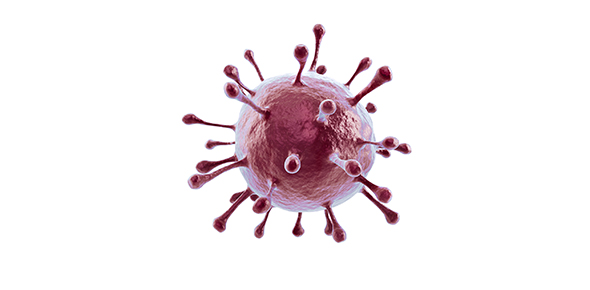Know The Cycle Of The Cell - The Science Quiz

10 Questions | Total Attempts: 227SettingsCell cycle is the name we give the process through which cells replicate and make two new cells. Cell cycle has different stages called G1, S, G2, and M. G1 is the stage where the cell is preparing to divide. To do this, it then moves into the S phase where the cell copies all the DNA. Know the cycle of the cell in this Science Quiz.

• 1.
The first stage of the cell cycle is
• A.

Interpahse

• B.

Mitosis

• C.

Meiosis

• D.

Anaphase

• E.

None of the above

• 2.
DNA is found in the nucleus on
• A.

The centromere

• B.

The nucleus

• C.

The chromatin

• D.

Vacuole

• E.

None of the above

• 3.
The genetic material doubles in
• A.

Interphase

• B.

Metaphase

• C.

Anaphase

• D.

Telophase

• E.

None of the above

• 4.
• A.

Interphase

• B.

Cytokinesis

• C.

DNA

• D.

Telophase

• E.

None of the above

• 5.
The end result of mitosis is ________ new cells
• A.

One

• B.

Two

• C.

Three

• D.

Four

• E.

None of the above

• 6.
The major purpose of mitosis is for growth and to replace worn out cells.
• A.

True

• B.

False

• 7.
_________ is a process where a single cell divides into two identical daughter cells (cell division).
• 8.
_______ is when the DNA in the cell is copied in preparation for cell division, this results in two identical full sets of chromosomes?.
• 9.
Each chromosome is composed of two sister chromatids, containing identical genetic information.
• A.

True

• B.

False

• 10.
At each pole of the cell a full set of chromosomes gather together at the stage of
• A.

Anaphase

• B.

Telophase

• C.

Metaphase

Related TopicsBack to top